NCERT Solutions for Class 6 Maths Chapter 13 Symmetry Ex 13.3 are part of NCERT Solutions for Class 6 Maths. Here we have given NCERT Solutions for Class 6 Maths Chapter 13 Symmetry Ex 13.3.

 Board CBSE Textbook NCERT Class Class 6 Subject Maths Chapter Chapter 13 Chapter Name Symmetry Exercise Ex 13.3 Number of Questions Solved 3 Category NCERT Solutions

## NCERT Solutions for Class 6 Maths Chapter 13 Symmetry Ex 13.3

Question 1.
Find the number of lines of symmetry in each of the following shapes. How will you check your answers?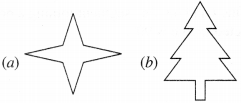Solution :
(a)Number of lines of symmetry = 4
(b)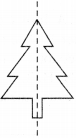Number of lines of symmetry = 1
(c)Number of lines of symmetry = 2
(d)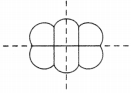Number of lines of symmetry = 2
(e)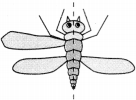Number of lines of symmetry = 1
(f)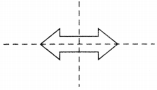Number of lines of symmetry = 2

Question 2.
Copy the following drawing on squared paper. Complete each one of them such that the resulting figure has the two dotted lines as two lines of symmetry :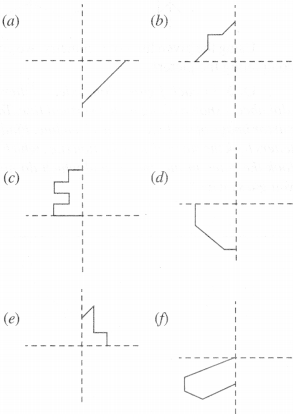How did you go about completing the picture?
Solution :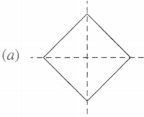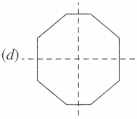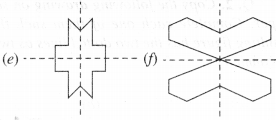Using the given lines of symmetry, we go about completing the picture.

Question 3.
In each figure alongside, a letter of the alphabet is shown along with a vertical line. Take the mirror image of the letter in the given line. Find which letters look the same after reflection (i.e., which letters look the same in the image) and which do not. Can you guess why?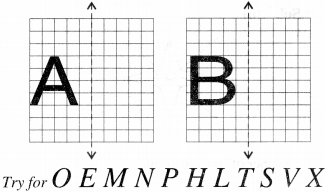Solution :
The letter A looks the same after reflection but not the letter B. The reason is that in reflection, the sense of direction changes. In the given letters, the letters O, M, N, H, T, V, and X look the same after reflections because these letters have a vertical line of symmetry.

We hope the NCERT Solutions for Class 6 Maths Chapter 13 Symmetry Ex 13.3 help you. If you have any query regarding NCERT Solutions for Class 6 Maths Chapter 13 Symmetry Ex 13.3, drop a comment below and we will get back to you at the earliest.